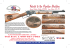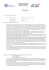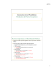# CMSC 411 : Homework #3

## Transcription

CMSC 411 : Homework #3
```CMSC 411 : Homework #3
Due on Tuesday, March 24, 2015
3:30pm
Anwar Mamat
1
Anwar Mamat
CMSC 411 ( 3:30pm): Homework #3
Problem 1
Pipeline
1. Use the following code fragment:
Listing 1: Code Fragment
5
Loop: LD
SD
DSUB
BNEZ
R1,0(R2)
R1,R1,#1
0(R2),R1
R2,R2,#4
R4,R3,R2
R4,Loop
;
;
;
;
;
;
R1=R1+1
R2=R2+4
R4=R3-R2
branch to loop if R4!=0
Assume that the initial value of R3 is R2 + 396 (i.e., 99 loop iterations).
Use the classic MIPS five-stage integer pipeline and assume all memory accesses take 1 clock cycle.
1. Using the following pipeline timing table, show the timing of this instruction sequence for the MIPS
pipeline without any forwarding or bypassing hardware but assuming a register read and a write in the
same clock cycle “forward” through the register file (e.g., value of R1 written by LD’s WB in 1st half
of cycle 5 can be read by DADDI’s ID in 2nd half of cycle 5). If all memory references take 1 cycle,
how many cycles does this loop take to execute? (20 points)
Figure 1:
2. Show the timing of this instruction sequence for the MIPS pipeline with normal forwarding and bypassing hardware. Assume that the branch is handled by predicting it as not taken. If all memory
references take 1 cycle, how many cycles does this loop take to execute? (20 points)
Figure 2:
3.
Page 2 of 3
Anwar Mamat
CMSC 411 ( 3:30pm): Homework #3
Problem 1
Problem 2
Suppose that in the original MIPS implementation (no pipeline), the stages to execute an instruction could
run this fast:
Control Signal
Value
IF
ID
EX
MEM
WB
15ns
8ns
7ns
15ns
5ns
1. How long, in nanoseconds, would it take to complete 100 instructions, consisting of 20 loads, 20 stores,
10 branches, and 50 register-register ALU instructions? Assume only loads and stores use the MEM
stage to execute. (10 points)
2. Suppose MIPS was implemented on a 5-stage pipeline with the same stages as above. If there are no
stalls in the pipeline, how many nanoseconds would it take to complete the same 100 instructions? (10
points)
3. Suppose that half of the loads cause a 3 cycle delay in the pipeline. How many nanoseconds would it
take to complete the 100 instructions? (10 points)
Problem 3
Suppose the branch frequencies (as percentages of all instructions) are as follows:
Conditional branches
Jumps and calls
Taken conditional branches
Not Taken conditional branches
15%
1%
60% (60% of the 15% branches)
40% (40% of the 15% branches)
1. We are examining a four-deep pipeline (assume the four stages of the pipeline are Instruction Fetch,
Instruction Decode, Execute, and Write Back, abbreviated IF, ID, EX, and WB, respectively) where
the branch is resolved at the end of the second cycle for unconditional branches and at the end of
the third cycle for conditional branches. Assuming that only the first pipe stage can always be done
independent of whether the branch goes and ignoring other pipeline stalls, how much faster would the
machine be without any branch hazards? (10 points)
2. Now assume a high-performance processor in which we have a 15-deep pipeline where the branch is
resolved at the end of the fifth cycle for unconditional branches and at the end of the tenth cycle for
conditional branches. Assuming that only the first pipe stage can always be done inde- pendent of
whether the branch goes and ignoring other pipeline stalls, how much faster would the machine be
without any branch hazards? (10 points)
What to submit
Submit a printed hardcopy of your solution before class starts at 3:30pm on 03/24/2015.
Page 3 of 3
```

### Empalme Gas Pipeline Branch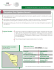### Hermosillo Gas Pipeline Branch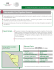### Introduction to Computer Architecture Assignment 2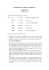### Samalayuca – Sasabe Gas Pipeline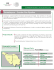### The Year in Review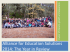### S. Ellis - Community Advisory Boards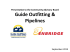### Colombia – Escobedo Gas Pipeline Geographic Location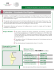### Wampee Conference Center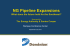### flyer - Centennial Classic Arms